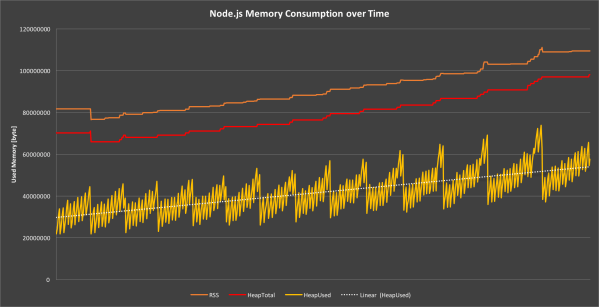# js递归函数造成的内存溢出(堆栈溢出)问题的解决方法

JAVA 7911浏览```function isEven (num) {
if (num === 0) {
return true;
}
//业余草：www.xttblog.com
if (num === 1) {
return false;
}
//业余草：www.xttblog.com
return isEven(Math.abs(num) - 2);
}
//Outputs: true
console.log(isEven(10));
//Outputs: false
console.log(isEven(9));```

```//不同的javascript引擎报错可能不同
//Outputs: Uncaught RangeError: Maximum call stack size exceeded
console.log(isEven(10000));```

## 使用闭包

```function isEven (num) {
if (num === 0) {
return true;
}
if (num === 1) {
return false;
}
//业余草：www.xttblog.com
return function() {
return isEven(Math.abs(num) - 2);
}
}
//Outputs: true
console.log(isEven(4)()());```

## 优化调用

```function isEven (num) {
if (num === 0) {
return true;
}
if (num === 1) {
return false;
}
return function() {
return isEven(Math.abs(num) - 2);
}
}
function trampoline (func, arg) {
var value = func(arg);
while(typeof value === "function") {
value = value();
}
return value;
}
//Outputs: true
console.log(trampoline(isEven, 10000));
//业余草：www.xttblog.com
//Outputs: false
console.log(trampoline(isEven, 10001));```